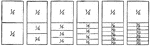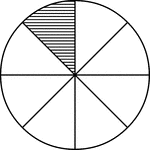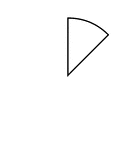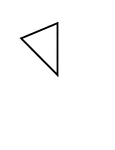### Fraction Comparisons

Rectangles comparing fraction values to one half: 1/4, 1/6, 1/8, 1/10, and 1/12.### Fraction Pie Divided into Eighths

A circle divided into eighths with one eighth shaded.### One Eighth of a Fraction Pie

One eighth of a circle.### Fractions of 8-sided Polygon

1/8 of a 8 sided polygon